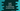# Three different Swift programs to check if a dictionary contains a key or not## Three different ways to check if a swift dictionary contains a key :

Dictionaries are collection with key-value pairs. It is like a hash table. We can access its elements using the key. Using a key, we can access its value.

Using the key, we can update, delete or modify its value. In this post, I will show you different ways to check if a swift dictionary contains one key or not.

## Method 1 : check if the value is nil :

Let’s consider the below example :

``````var given_dict = ["one": 1, "two": 2, "three": 3]

print(given_dict)

given_dict["two"] = nil

print(given_dict)``````

given_dict is a dictionary with three key-value pairs. We are assigning the value of key two to nil. It gives the below output :

``````["one": 1, "two": 2, "three": 3]
["one": 1, "three": 3]``````

As you can see, if we assign any value nil, it removes that pair. So, we can’t have any key with value equal to nil.

We can use this logic to check if a dictionary contains a specific key or not. For example :

``````var given_dict = ["one": 1, "two": 2, "three": 3]

if(given_dict["one"] != nil){
print("Key 'one' is found in the dictionary")
}else{
}

if(given_dict["four"] != nil){
print("Key 'four' is found in the dictionary")
}else{
}``````

Here, we have two if-else blocks to check if given_dict contains one and four as key or not. It will print the below output :

``````Key 'one' is found in the dictionary

## Method 2: Using if let :

We can also use if let to safely unwrap and check if a key exists :

``````var given_dict = ["one": 1, "two": 2, "three": 3]

if let _ = given_dict["one"]{
print("Key 'one' is found in the dictionary")
}else{
}

if let _ = given_dict["four"]{
print("Key 'four' is found in the dictionary")
}else{
}``````

It will print the same output.

## Method 3: Using index(forKey: key) :

index(forKey: key) method is used to get the index of a dictionary key. If the key is not found, it will return nil. The below example uses index to check if a key exists or not in a dictionary :

``````var given_dict = ["one": 1, "two": 2, "three": 3]

if(given_dict.index(forKey: "one") != nil){
print("Key 'one' is found in the dictionary")
}else{